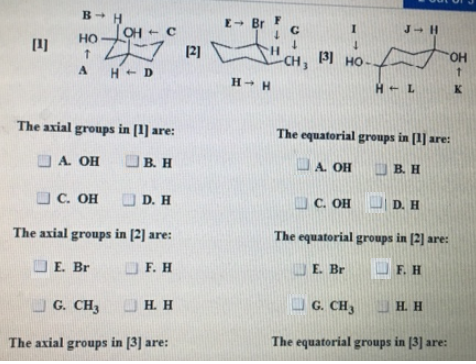# Problem: The axial groups in  are: The equatorial groups in  are: The axial groups in  are: The equatorial groups in  are: The axial groups in  are: The equatorial groups in  are:

###### FREE Expert Solution###### Problem Details

The axial groups in  are:

The equatorial groups in  are:

The axial groups in  are:

The equatorial groups in  are:

The axial groups in  are:

The equatorial groups in  are: